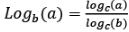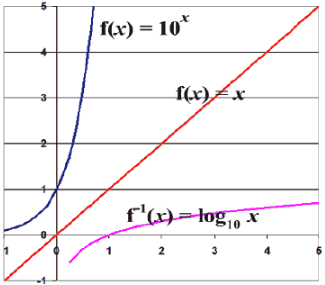Courses

# Important Formulae: Logarithms CAT Notes | EduRev

## Quant : Important Formulae: Logarithms CAT Notes | EduRev

The document Important Formulae: Logarithms CAT Notes | EduRev is a part of the Quant Course Quantitative Aptitude (Quant).
All you need of Quant at this link: Quant

Logarithm

• Log(ab) = Log(a) + Log(b)
• Log(a/b) = Log(a) - Log(b)
• Log(an) = nLog(a)
•• Logb b = 1
• Logb 1 = 0
• Logb bx = x
• Ln x means loge x
• x = blogbxOffer running on EduRev: Apply code STAYHOME200 to get INR 200 off on our premium plan EduRev Infinity!

## Quantitative Aptitude (Quant)

116 videos|131 docs|131 tests

,

,

,

,

,

,

,

,

,

,

,

,

,

,

,

,

,

,

,

,

,

;